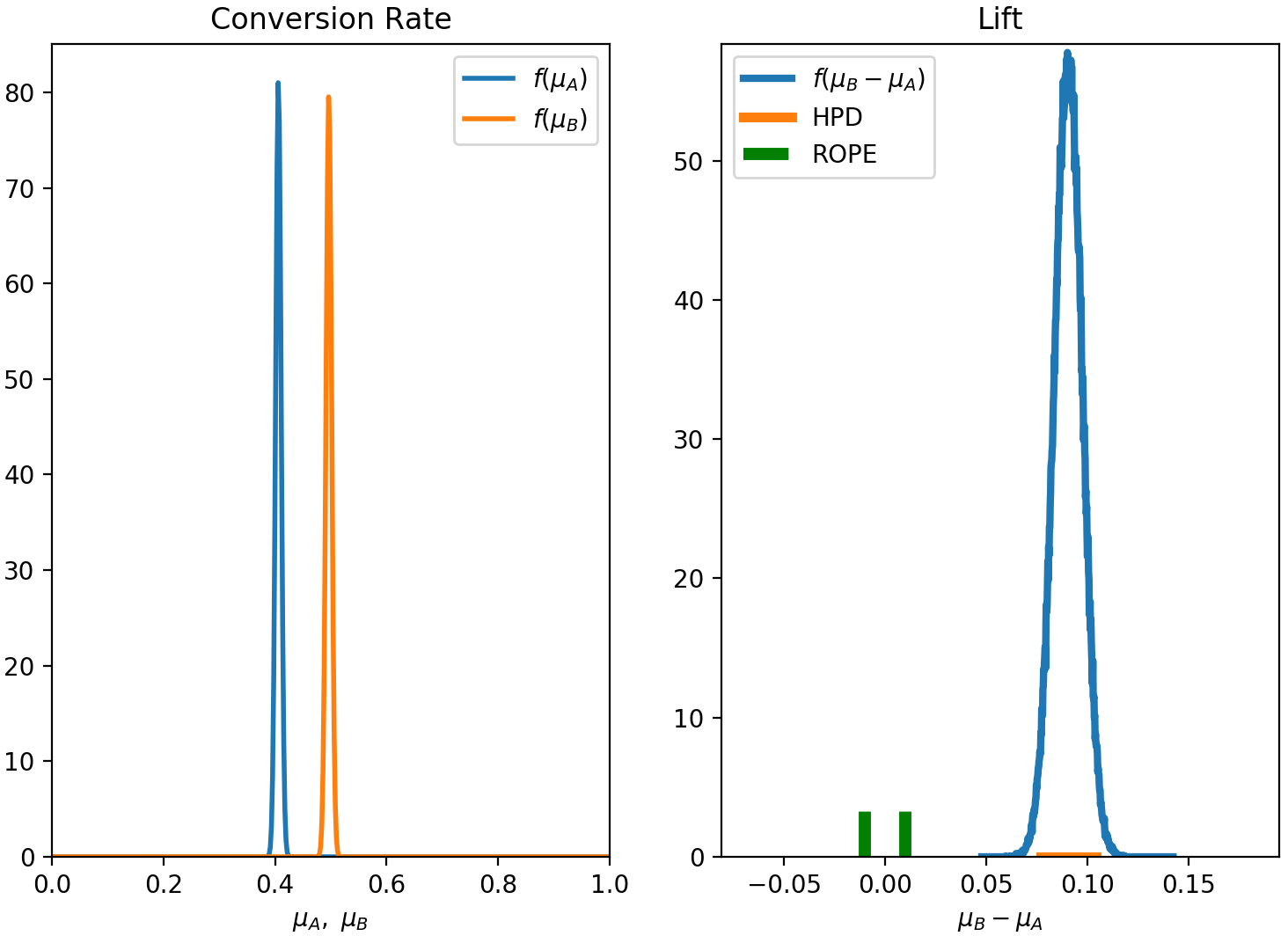/ abyes Public

A Python package for Bayesian A/B Testing

# cbellei/abyes

Switch branches/tags
Nothing to show

A tag already exists with the provided branch name. Many Git commands accept both tag and branch names, so creating this branch may cause unexpected behavior. Are you sure you want to create this branch?

## Files

Failed to load latest commit information.
Type
Name
Commit time

# aByes

aByes is a Python package for Bayesian A/B Testing, which supports two main decision rules:

A lot of the underlying theory is discussed in this blog post.

## Installation

• In your target folder, clone the repository with the command:

`git clone https://github.com/cbellei/abyes.git`
• Then, inside the same folder (as always, it is advisable to use a virtual environment):

`pip install .`
• To check that the package has been installed, in the Python shell type:

`import abyes`
• If everything works correctly, the package will be imported without errors.

## Dependencies

• aByes is tested on Python 3.5 and depends on NumPy, Scipy, Matplotlib, Pymc3 (see `requirements.txt` for version

information).

## How to use aByes

The main steps to run the analysis of an A/B experiment are:

• Aggregate the data for the "A" and "B" variations in a List of numpy arrays
• Decide how to do the analysis. Options are: 1. analytic solution; 2. MCMC solution (using PyMC3); 3. compare the analytic and MCMC solutions
• Set decision rule. Options are: 1. ROPE method; 2. Expected Loss method
• Set parameter to use for the decision. Options are: 1. Lift (difference in means); 2. Effect size

These and many more examples and instructions can be found in this blogpost.

## Example

• In IPython, type:

```import abyes as ab
import numpy as np

data = [np.random.binomial(1, 0.4, size=10000), np.random.binomial(1, 0.5, size=10000)]
exp = ab.AbExp(method='analytic', decision_var = 'lift', rule='rope', rope=(-0.01,0.01), plot=True)
exp.experiment(data)```
• This will plot the posterior distribution:• It will then give the following result:

```*** abyes ***

Method = analytic
Decision Rule = rope
Alpha = 0.95
Rope = (-0.01, 0.01)
Decision Variable = lift

Result is conclusive: B variant is winner!```
• There are many more examples available in the file `example.py`, which can be run from the root directory with the command:

`python abyes/examples/examples.py`

## Limitations

Currently, aByes:

• only focuses on conversion rate experiments
• allows for only two variants at a time to be tested

These shortcomings may be improved in future versions of aByes. (Feel free to fork the project and make these improvements yourself!)

## Licence

A Python package for Bayesian A/B Testing

## Releases

No releases published

## Packages 0

No packages published

•
•